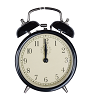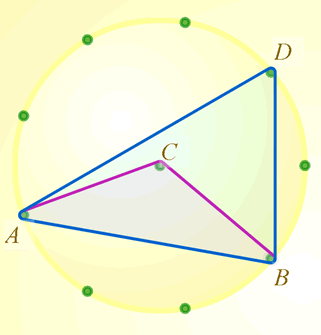#### You may also like### On Time

On a clock the three hands - the second, minute and hour hands - are on the same axis. How often in a 24 hour day will the second hand be parallel to either of the two other hands?### Estimating Angles

How good are you at estimating angles?### LOGO Challenge 1 - Star Square

Can you use LOGO to create this star pattern made from squares. Only basic LOGO knowledge needed.

# Subtended Angles

##### Age 11 to 14Challenge Level

In this problem you can make use of the interactivity below.

Choose two points on the edge of the circle. Call them $A$ and $B$.

Join these points to the centre, $C$. What is the angle at $C$?

Join $A$ and $B$ to a point on the edge. Call that point $D$. What is the angle at $D$?

What do you notice?Would the same thing happen if $D$ took a different position on the edge of the circle?

Would the same thing happen if you started with a different two points on the edge of the circle?

Would the same thing happen if you started with any two points on the edge of any circle?
Can you prove it?

For printable sets of circle templates for use with this activity, please see Printable Resources page.

Many thanks to Geoff Faux who introduced us to the merits of the 9 pin circular geo-board.

The boards, moulded in crystal clear ABS that can be used on an OHP (185 cm in diameter), together with a teacher's guide, are available from Geoff at Education Initiatives